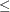### Variable and Constraint Status

Upon termination of the LP solver, the .status suffix of each decision variable and constraint stores information about the status of that variable or constraint. For more information about suffixes in the OPTMODEL procedure, see the section Suffixes.

#### Variable Status

The .status suffix of a decision variable specifies the status of that decision variable. The suffix can take one of the following values:

B

basic variable

L

nonbasic variable at its lower bound

U

nonbasic variable at its upper bound

F

free variable

S

superbasic variable (a nonbasic variable with a value strictly between its bounds)

I

LP model infeasible (all decision variables have .status equal to I)

For the interior point solver with IIS= OFF, .status is blank.

The following values can appear only if IIS= ON. See the section Irreducible Infeasible Set for details.

I_L

the lower bound of the variable is violated

I_U

the upper bound of the variable is violated

I_F

the fixed bound of the variable is violated

#### Constraint Status

The .status suffix of a constraint specifies the status of the slack variable for that constraint. The suffix can take one of the following values:

B

basic variable

L

nonbasic variable at its lower bound

U

nonbasic variable at its upper bound

F

free variable

S

superbasic variable (a nonbasic variable with a value strictly between its bounds)

I

LP model infeasible (all decision variables have .status equal to I)

The following values can appear only if option IIS= ON. See the section Irreducible Infeasible Set for details.

I_L

the GE () condition of the constraint is violated

I_U

the LE () condition of the constraint is violated

I_F

the EQ (=) condition of the constraint is violated